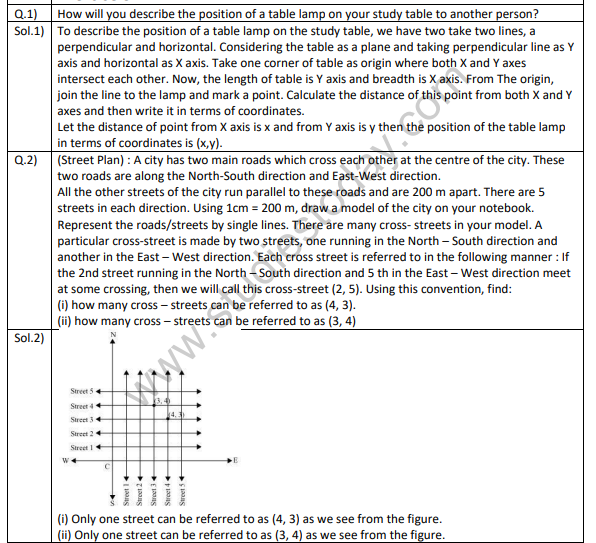# NCERT Solutions Class 9 Mathematics Chapter 3 Coordinate Geometry

## Chapter 3 Coordinate Geometry Class 9 NCERT Solutions

Class 9 Mathematics students should refer to the following NCERT questions with answers for Chapter 3 Coordinate Geometry in standard 9. These NCERT Solutions with answers for Grade 9 Mathematics will come in exams and help you to score good marks

### Chapter 3 Coordinate Geometry NCERT Solutions Class 9

NCERT Solutions class 9 Mathematics Chapter 3 Coordinate Geometry. NCERT book for Mathematics in class 9 is strongly recommended by teachers and the CBSE and NCERT boards. The NCERT books are made as per syllabus issued by CBSE and covers all topics which will come in exams. The students should understand the concepts given in the books and solve the questions given at the end of each chapter. You can download the NCERT solutions for class 9 Mathematics free in PDF made by teachers of the best schools in India from studiestoday.com from the link provided below and can be downloaded in pdf format for free. You can also get free study material for class 9 by clicking links below.## Books recommended by teachers

 NCERT Solutions Class 9 Mathematics Chapter 1 Number Systems
 NCERT Solutions Class 9 Mathematics Chapter 2 Polynomials
 NCERT Solutions Class 9 Mathematics Chapter 3 Coordinate Geometry
 NCERT Solutions Class 9 Mathematics Chapter 4 Linear Equations In Two Variables
 NCERT Solutions Class 9 Mathematics Chapter 5 Introduction to Euclid's Geometry
 NCERT Solutions Class 9 Mathematics Chapter 6 Lines and Angles Variables
 NCERT Solutions Class 9 Mathematics Chapter 7 Triangles
 NCERT Solutions Class 9 Mathematics Chapter 8 Quadrilaterals
 NCERT Solutions Class 9 Mathematics Chapter 9 Areas of Parallelograms and Triangles
 NCERT Solutions Class 9 Mathematics Chapter 10 Circles
 NCERT Solutions Class 9 Mathematics Chapter 11 Construction
 NCERT Solutions Class 9 Mathematics Chapter 12 Herons Formula
 NCERT Solutions Class 9 Mathematics Chapter 13 Surface Area and Volume
 NCERT Solutions Class 9 Mathematics Chapter 14 Statistics
 NCERT Solutions Class 9 Mathematics Chapter 15 Probability